Today we are going to talk about the most important concept in statistics: the Central Limit Theorem (CLT). It is usually called the most important theorem in statistics.

We all know we can’t sample all population – otherwise we don’t need all those inferential tools. We can only sample a finite number of elements from any population, and then hope that our descriptive statistics from this smaller sample will be close enough to the population. However, “close enough” is not enough. We need to be more specific. Well, we’ll need the Central Limit Theorem to give us a more exact estimate of how close are we to the population estimate.

I’ll repeat this: we only have a small sample (say 300 voters), and based on this small sample, we can claim that 20% will vote for candidate X or approves the president. Someone might yell “Seriously? How dare they talk about ALL THE MILLIONS OF AMERICAN VOTERS based on making few hundred calls?”. It is CTL that makes all that possible.

Let’s make this more concrete by thinking of CLT through simulations. Here, we will first make a population (so that we know the true estimates such as the mean and the standard deviation), and then we’ll sample from this population and see how close can we get.

Remember from last lab, we saw that the mean is called an unbiased estimate because it approximates the population mean (of a normal distribution) even in low number of samples. However, the standard deviation is called a biased estimate because it is always below the population standard deviation – and the more you sample (larger N), the closer you get to the population mean. Here, we will ask: then how should we estimate the variability of the population if our estimate from the sample (i.e., the standard deviation) is always biased?

# Sampling distribution of the mean

To explore those answers, we will first make a population with known parameters (mean and standard deviation) and then we will repeatedly sample from this population to record the means.

First let’s make a population of size 100 scores from a normal distribution of mean = 100 and a standard deviation = 15

sd_uncorrected<-function(x){
return(sqrt(sum((x-mean(x))^2)/length(x)))
}

population <- rnorm(n = 100, mean = 100, sd = 15)
population_mean <- mean(population)
population_std  <- sd_uncorrected(population)

Let’s print those estimates:

paste('population mean = ', population_mean)
##  "population mean =  98.6224312567792"
paste('population std  = ', population_std)
##  "population std  =  14.5010092891134"

Now, in any study, we are not going to sample all the population. We can only sample a finite subset of that population. We will see what happens when we sample a small number of observations and examine its mean:

sample_size <- 10 # how many elements we want to sample
sample_n <- sample(population, size = sample_size, replace = FALSE)
sample_n
##    70.93210 108.24827 105.45649 130.37193  87.73431 105.17426  81.29932
##   114.51379 120.74231 123.28389

Based on what we learned from the last lab, we know that the mean should be close enough to the population mean (which is 100), but the standard deviation will be less than the standard deviation of the population (which is 15).

mean(sample_n)
##  104.7757
sd_uncorrected(sample_n)
##  18.29509

So our sample estimates are quite different from the population parametres. The central limit theorem tells us that if you sample many more, and record the mean of those samples, then the distribution of those means should approximate a normal distribution. I know this does not sound that exciting at the moment, but let’s see that normal distribution first. We will now take many samples, and record the means of those samples, and then we will make a histogram of those means.

n_experiments <- 1000  # we will sample 1000 times
sample_size <- 10  # how many elements we want to sample?
sample_means <- c()

for (i in 1:n_experiments) {
this_sample <- sample(population, size=sample_size, replace=FALSE)
sample_means[i] <- mean(this_sample)
}

Now we will plot those means:

library(ggplot2)
sample_means_df <- data.frame(means=sample_means)
ggplot(sample_means_df, aes(x=means)) +
geom_histogram() +
geom_vline(xintercept = population_mean, color='red') + # population mean
geom_vline(xintercept = mean(sample_means_df$means), color='black')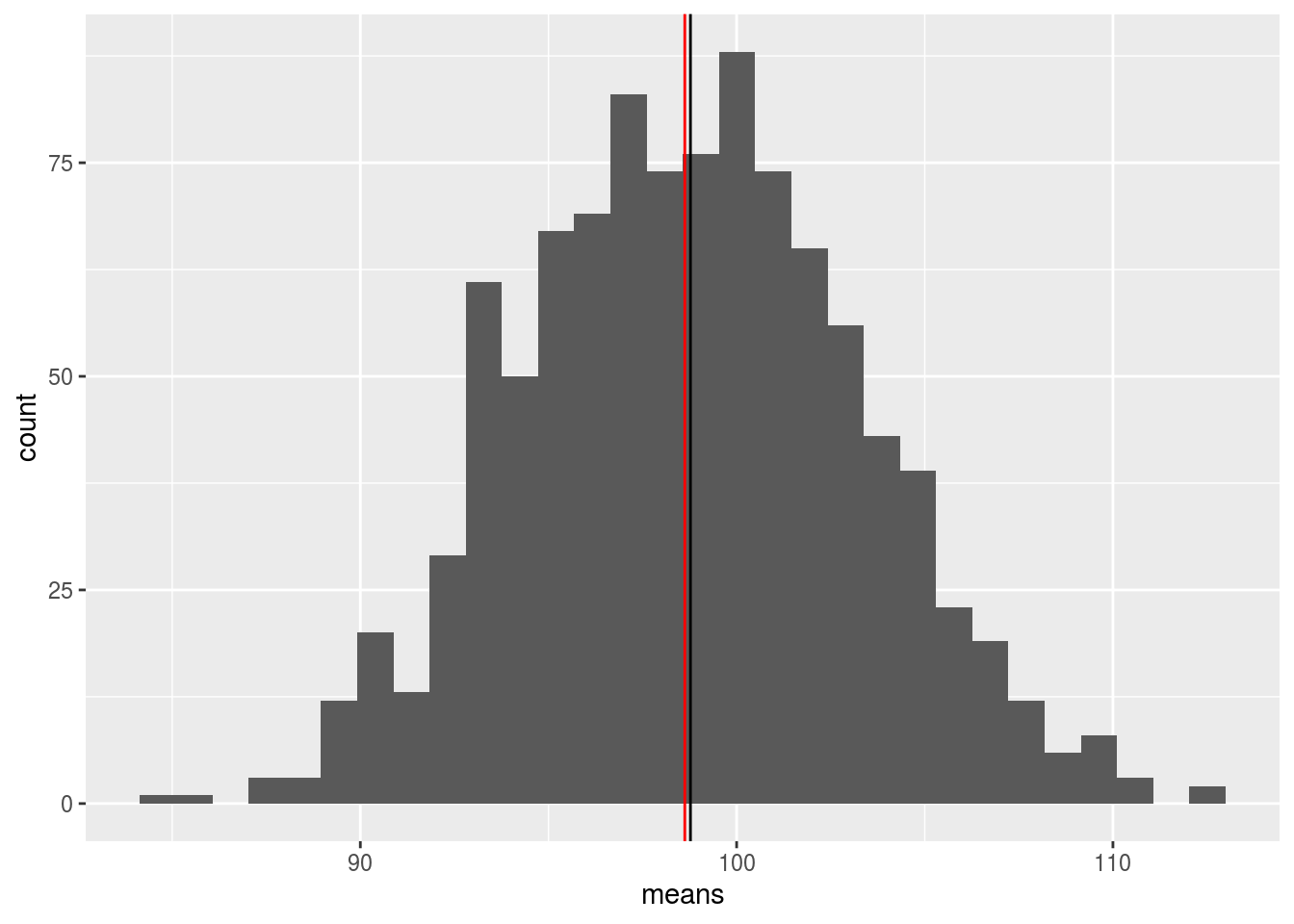Look at that! First, you see that it is a bell-curve (i.e., a normal distribution). But remember, those are not the actual samples! those are the means of those repeated samples: so we should expect that the mean of the sample means (colored black) is close enough to our population mean (colored red). Also, note that the numbers are pretty narrow around the mean of the means. We can actually compute the standard deviation: sd_uncorrected(sample_means_df$means)
##  4.486699

The standard deviation of the sampling distribution of the means is a very important quantity: it is called the standard error of the mean. (mean here refers to the mean of sample means and NOT mean of a given sample).

Now, this number is VERY helpful. Why? Because we can use that standard deviation to know what is the proportion of means that should lie within a given interval. For example, from last class, we know that if you have a normal distribution, then you can use pnorm to calculate the proportion of samples below any value or between any two values. For example, in a z-distribution that has a mean of 0 and a standard deviation of 1, the proportion of values that lie between 1 and -1 is approximately 68% :

pnorm(q=1, mean=0, sd=1, lower.tail = TRUE) -
pnorm(q=-1, mean=0, sd=1, lower.tail = TRUE) 
##  0.6826895

meaning: 68% of values should lie within 1 standard deviation from the mean (either either below or above the mean). If you have the standard deviation of the mean of the means, then you can say things like: the means should be 1 standard deviation above or below this number in 68% of the time. In other words: you can actually use that standard deviation to quantify the variability of any estimate (e.g., the mean of the sample) and give a range of the possible values (e.g., 1 std above and below). Does that remind you of something?? HMMM.. Try Confidence Intervals!

There is only one tiny problem here. Since we created that population, and made all those repeated sampling, we already know what is the value of the standard error. However, in a real-world situation, you only have a sample from that unknown population. How should you calculate the standard error (or the standard deviation of the mean of the means)?

Believe it or not, you can do that using only the sample standard deviation and the sample size! The formula for the standard error of the mean is:

$\frac{sd_{sample}}{\sqrt{N}}$

Just divide your sample standard deviation with the square root of sample size! (well, here you should say wow). Let’s take a sample from the population and see if it is close to the value we got above:

sample_size <- 10
another_sample <- sample(population, size=sample_size, replace=FALSE)
sd(another_sample)/sqrt(sample_size)
##  5.668853

Which should be reasonably close. How close? well, that depends on the sample size! As the sample size increases, your samples will be much more representative of the population and thus your estimated mean of the means will be much closer to the population mean.

# Effect of sample size

Let’s run a simulation of how much sample size you need to approximate the true variability of the population. This time, we don’t really need to define a population: we only need to know that we are sampling from a population of mean equals to 100 and a standard deviation equals to 15. (or change those to your own values)

population_mean <- 100
population_std  <- 15
n_experiments <- 1000  # we will sample 1000 times
sample_size <- 1:100     # how many elements we want to sample?

mean_of_means <- c()
mean_of_sds <- c()
mean_of_sems_sampled <- c()

for (this_sample_size in sample_size) {
sample_means <- c()
sample_sds <- c()
sample_sems_true <- c()
sample_sems_sampled <- c()
for (i in 1:n_experiments) {
this_sample <- rnorm(n=this_sample_size, mean=population_mean, sd=population_std)
sample_means[i] <- mean(this_sample)
sample_sds[i] <- sd(this_sample)
sample_sems_sampled[i] <- sd(this_sample)/sqrt(this_sample_size)
}
mean_of_means[this_sample_size] <- mean(sample_means)
mean_of_sds[this_sample_size] <-mean(sample_sds)
mean_of_sems_sampled[this_sample_size] <-mean(sample_sems_sampled)
}

my_df <- data.frame(sample_size,
mean_of_means,
mean_of_sds,
mean_of_sems_sampled)
library(ggpubr)
g1 <- ggplot(my_df, aes(x=sample_size, y=mean_of_means)) +
#geom_point() +
geom_line() +
geom_hline(yintercept = population_mean) +
ylim(c(95, 105))
g2 <- ggplot(my_df, aes(x=sample_size, y=mean_of_sds)) +
#geom_point() +
geom_line() +
geom_hline(yintercept = population_std)
g3 <- ggplot(my_df, aes(x=sample_size)) +
#geom_point() +
geom_line(aes( y=mean_of_sems_sampled), color='black') +
geom_hline(yintercept = 0)

ggarrange(g1, g2, g3, ncol = 3, nrow = 1)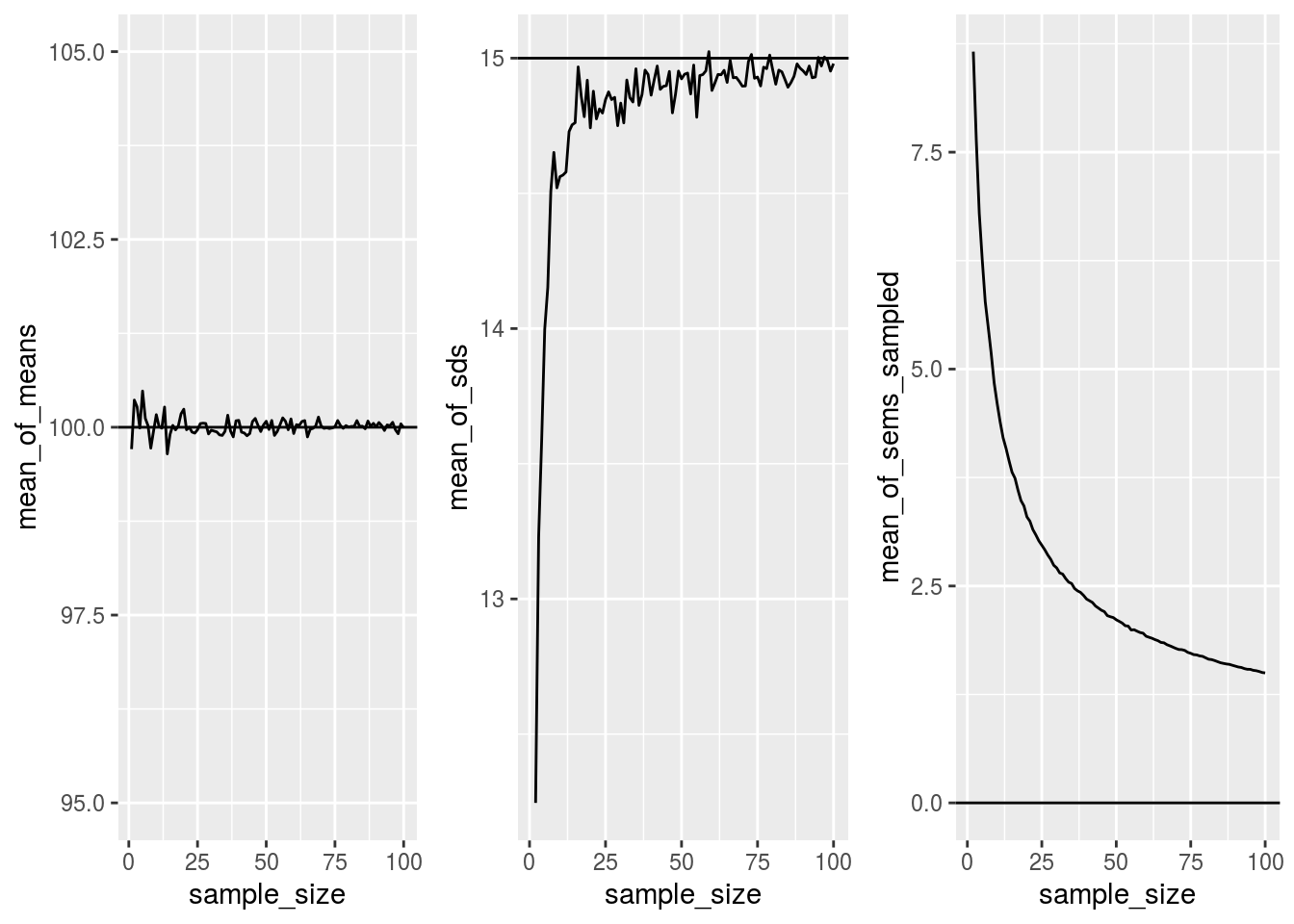# Sampling any statistics

The beauty is that it is not just the sample mean that approximate the normal distribution under the repeated sampling, it is actually any statistic. Here, we make a simulation of the median, max and min to see what happens to the sampling distribution of those statistics

all_df<-data.frame()
for(i in 1:1000){
my_sample<-rnorm(50,100,20)
sample_mean<-mean(my_sample)
sample_sd<-sd(my_sample)
sample_max<-max(my_sample)
sample_median<-median(my_sample)
t_df<-data.frame(i,sample_mean,sample_sd,sample_max,sample_median)
all_df<-rbind(all_df,t_df)
}

library(ggpubr)
a<-ggplot(all_df,aes(x=sample_mean))+
geom_histogram(color="white")+
theme_classic()
b<-ggplot(all_df,aes(x=sample_sd))+
geom_histogram(color="white")+
theme_classic()
c<-ggplot(all_df,aes(x=sample_max))+
geom_histogram(color="white")+
theme_classic()
d<-ggplot(all_df,aes(x=sample_median))+
geom_histogram(color="white")+
theme_classic()

ggarrange(a,b,c,d,
ncol = 2, nrow = 2)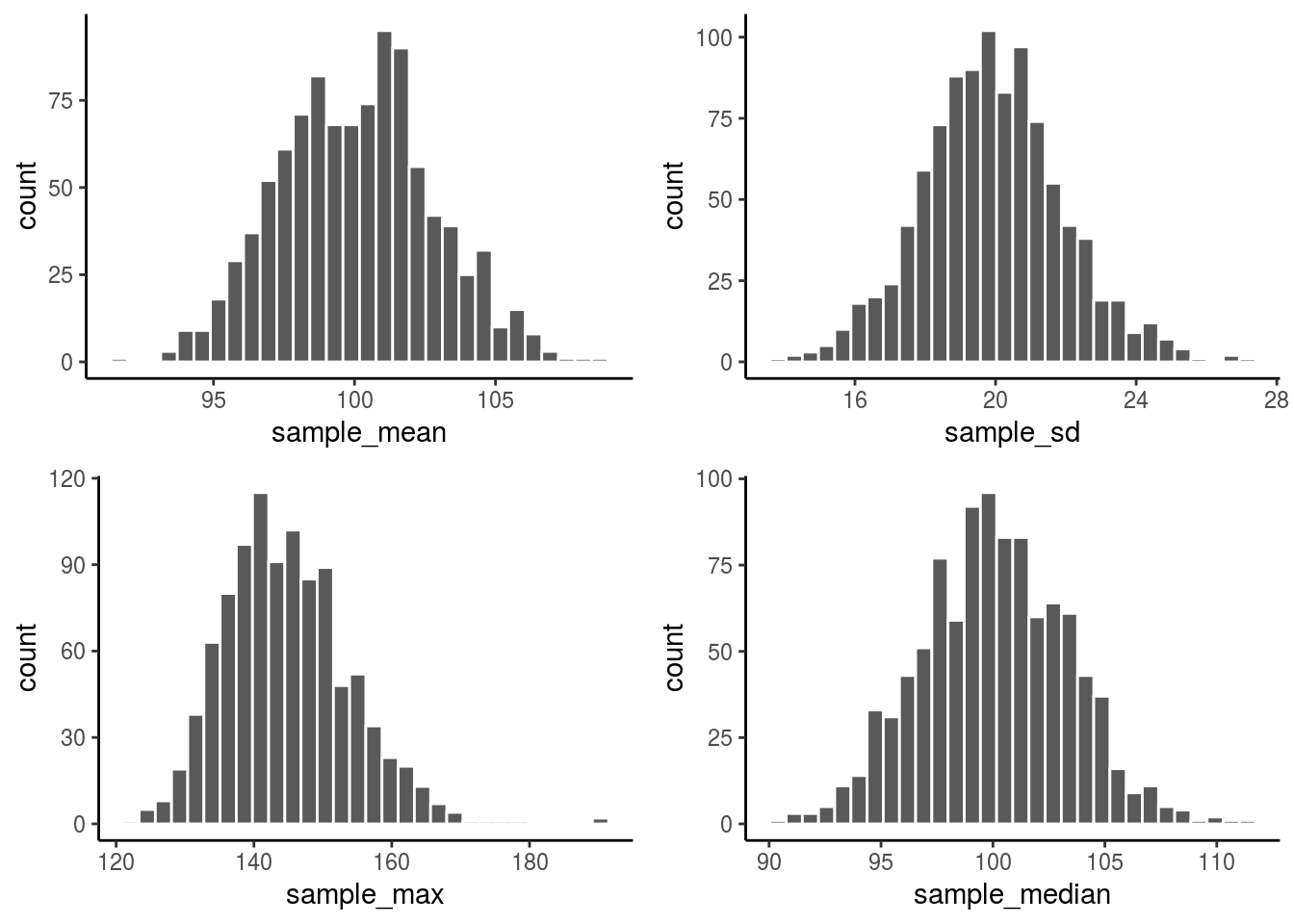More than that, you don’t even need normally distributed numbers to sample from. You can actually sample from ANY distribution and, under a larger N, you still get a normal distribution of the sampling distribution of the mean (but not necessarily for other statistics)! That should make you pause. This means that despite the underlying distribution of the things we want to study we still end up with a normal shape for the sampling distribution of the mean (given you have enough N). While you are at that pause, let’s make a simulation with a uniform distribution and see what happens:

all_df<-data.frame()
for(i in 1:1000){
my_sample<-runif(50,min=0,max=100)  # Here we changed the distribution of the samples
sample_mean<-mean(my_sample)
sample_sd<-sd(my_sample)
sample_max<-max(my_sample)
sample_median<-median(my_sample)
t_df<-data.frame(i,sample_mean,sample_sd,sample_max,sample_median)
all_df<-rbind(all_df,t_df)
}

library(ggpubr)
a<-ggplot(all_df,aes(x=sample_mean))+
geom_histogram(color="white")+
theme_classic()
b<-ggplot(all_df,aes(x=sample_sd))+
geom_histogram(color="white")+
theme_classic()
c<-ggplot(all_df,aes(x=sample_max))+
geom_histogram(color="white")+
theme_classic()
d<-ggplot(all_df,aes(x=sample_median))+
geom_histogram(color="white")+
theme_classic()

ggarrange(a,b,c,d,
ncol = 2, nrow = 2)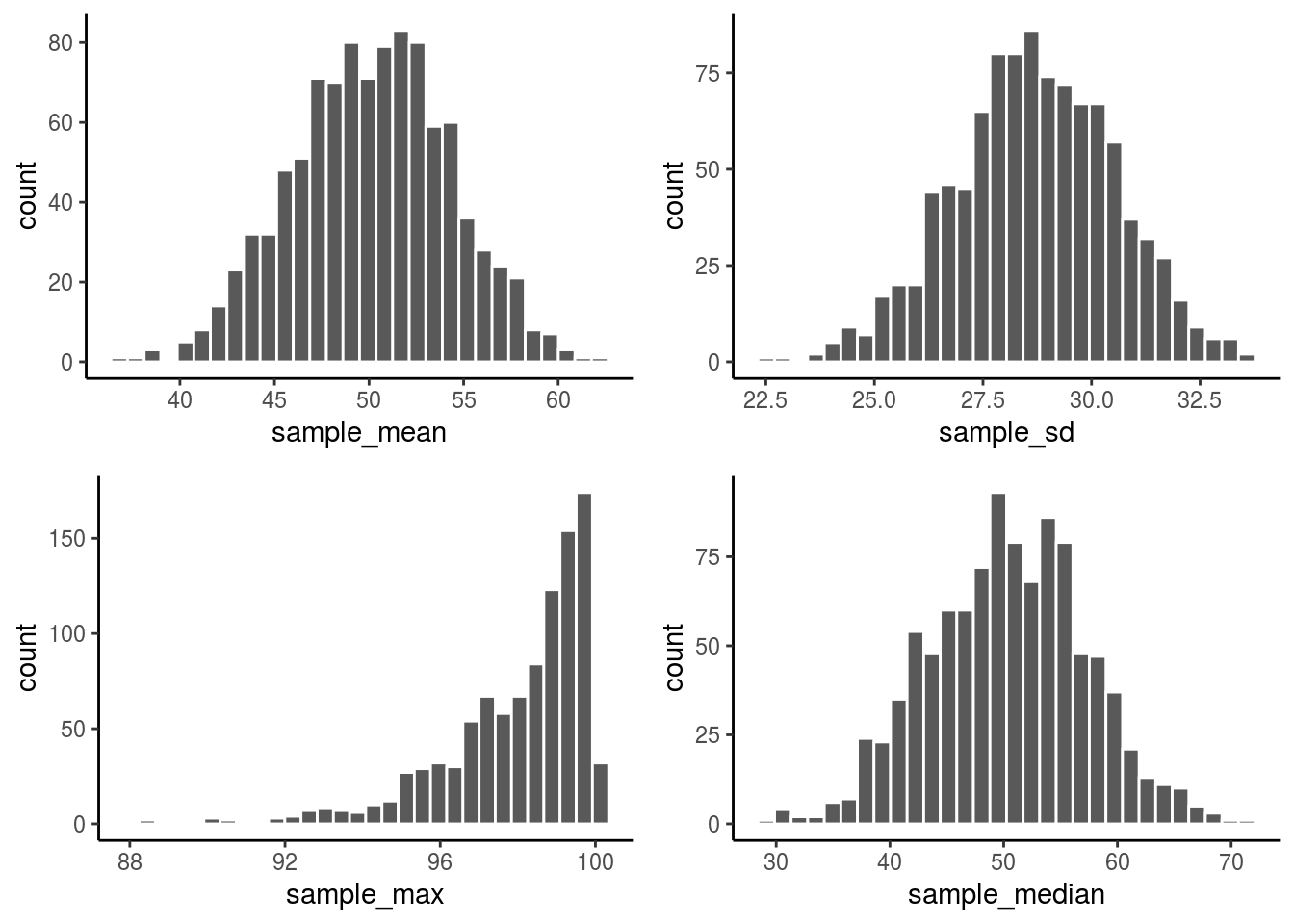See that except for the max, all other distributions are actually normal. Essentially this means that CTL gave us a green light to sample from any distribution out there and we’ll be assured that we can get to the mean of that distribution as N gets larger.

# The Confidence Intervals

Now that we know the concept of standard error (which is the standard deviation of the sampling distribution of the mean), and since we know that the means should look like a normal distribution (as you increase your N), we want to discuss how to use those insights to come up with a confidence interval for any estimate.

Let’s say that we sampled few people, and measured their heights. We got a mean of 5.5 feet and a standard deviation of 3. How can we use the standard error of the mean to come up with some quantity that reflects our confidence of that estimate. In other words, had I taken another random sample, how far my estimate should move? If I have taken many many samples, what is the most probable values of the average heights that I should observe, say, 95% of the time?

We already know the answers to all those questions! Given that the mean height of repeated sampling approximate a normal distribution, and given what we know about the normal distribution probabilities, we can devise a tool that tells us what is the required range of values that contains 68% of the possible means (around a given mean) – or any other percentage, this is just an example.

Remember, in a z-distribution, the mean is zero and the standard deviation is one. We can simply find the corresponding z-scores of the probabilities we want to use, and multiply those by the standard error of the mean.

$UpperLimit = \bar{x} + Z_{above}*SEM$ $LowerLimit = \bar{x} - Z_{below}*SEM$

For example, if we want to find the 68% confidence interval of a given sample mean, we simply calculate the z-score of an area .68 around the mean. Or, .34 above the mean and .34 below the mean (they should be equal)

#Remember, we have to subtract that area from half of the area
lower_z <- qnorm(p=.5-.34, mean=0, sd=1, lower.tail = TRUE)
upper_z <- qnorm(p=.5+.34, mean=0, sd=1, lower.tail = TRUE)

Which should give us those values:

c(lower_z, upper_z)
##  -0.9944579  0.9944579

meaning that we need to be ~1 standard deviations above or below the mean to include 68% of the values in that distribution. We should multiply those values by the SEM to give the confidence interval. How amazing is that? as the means of sampling means approzimate normal distribution, we just used the probabilities of the normal distribution to compute the bounds!

Let’s make one more simulation to see if those calculations are true. Here we will simulate a population, then sample from it and use the confidence intervals to test of the sampled mean values always within that interval:

population <- rnorm(n=100, mean=100, sd=15)
sample_n <- sample(population, size=10, replace=FALSE)
paste('mean of the sample is: ', mean(sample_n))
##  "mean of the sample is:  104.557724702423"
# calculate confidence interval
SEM <- sd(sample_n)/sqrt(length(sample_n))
upper_limit <- mean(sample_n) + 1 * SEM
lower_limit <- mean(sample_n) - 1 * SEM
paste('Upper limit is: ', upper_limit)
##  "Upper limit is:  108.559530220211"
paste('Lower limit is: ', lower_limit)
##  "Lower limit is:  100.555919184635"

Do the upper limit and the lower limit surround the population mean (here is 100)? You might want to run the previous cell many times to see how many times you see it within or outside the interval. Remember, those calculations say that 68% of the time you should observe the mean within those bounds. Let’s a lot more samples and calculate the times we are within those bounds (do they equal to 68%?):

sample_means <- c()
sample_sds <- c()
sample_size <- 50
n_experiments <- 100
for (i in 1:n_experiments) {
sample_n <- rnorm(n=sample_size, mean=100, sd=15)
sample_means[i] <- mean(sample_n)
sample_sds[i] <- sd(sample_n)
}
SEM <- sample_sds/sqrt(sample_size)
sample_upper_limit <- sample_means + 1 * SEM
sample_lower_limit <- sample_means - 1 * SEM

Now, let’s make a ggplot that have the means, upper limits and lower limits as lines:

my_df<- data.frame(n=1:n_experiments,
means=sample_means,
lower_limit=sample_lower_limit,
upper_limit=sample_upper_limit)
ggplot(my_df, aes(x=n, y=means))+
geom_segment(aes(y=lower_limit, yend=upper_limit, x=n, xend=n))+
geom_point(color='red') +
geom_point(aes(y=lower_limit)) +
geom_point(aes(y=upper_limit)) +
geom_hline(yintercept = 100)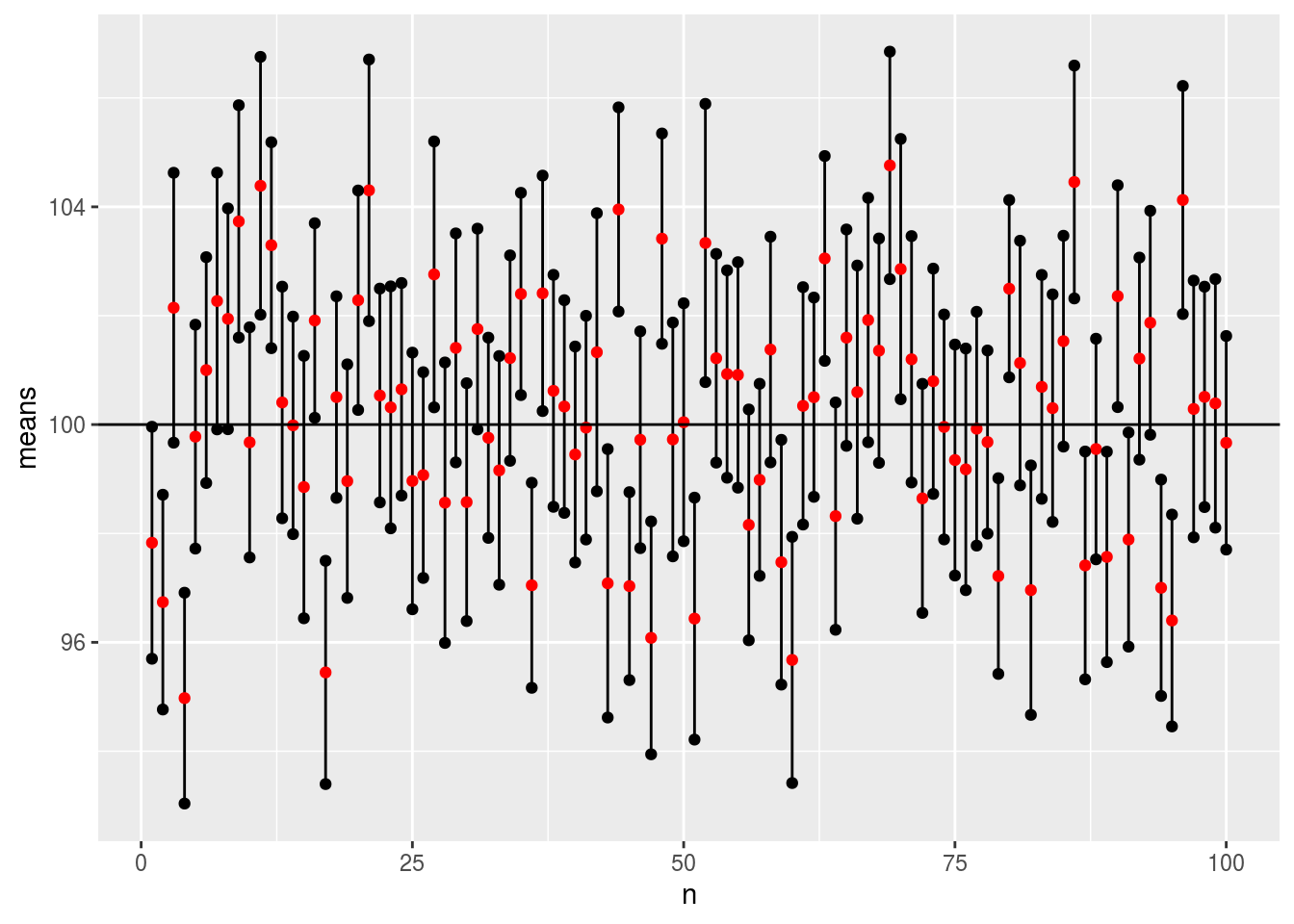Now, we should expect that only 68% of the lines contain the true population mean (which is 100).

mean(100 < my_df$upper_limit & 100 > my_df$lower_limit)
##  0.63

That was a feat. The value might be a little bit above or below .68, but try increasing the number of sampling trials (i.e., n_experiments) and you should expect the value to be closer and closer to .68.

# Recap:

What is the Central Limit Theorem ?

1. If you make samples from any distribution and record their means (or any statistics), then the means of those samples should approximate a normal distribution (provided you have large N)

2. The mean of the sampling distribution of the means (or the mean of the means) should approximate the true population mean from which those samples are drawn from (provided you have a large N)

3. The larger the sample size that is drawn, the more accurate you will be to the normal distribution and the smaller is the standard deviation of the sampling distribution of the mean.

I hope that you now understand why it makes sense to call a few hundred voters to estimate the % of AMERICAN PEOPLE voting for candidate X. Or more importantly, why do we only ask a few subjects (either humans or non-human samples) and generalize those observations to reflect the true state of the world. However, those samples MUST BE randomly sampled from the population. All the three points above rely on the fact that those samples are randomly sampled.

# Exercises

• Explain the concept of sampling error.
• Explain why the standard deviation of the sampling distribution of mean gets smaller as sample size increases.
• Make a simulation that shows how the SEM form a sample changes as you change the standard deviation of the population (modify the code above; do not change other parameters).
• Let’s say I know that my estimate of a given mean is very close to 50 and the standard deviation is 20 (based on previous studies). Make a simulation that shows me how the SEM changes as a function of sample size (go from 1 to 100).
• In the confidence interval simulations, what happens if you sample from a very small sample sizes? (change sample_size to some small number like 2 and re-run those two cells without changing anything – what do you notice in the plot? You don’t need to paste the code, just re-run the code multiple times)